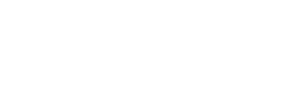kg

### 42AT10/15-2 Aluminium Pulley

• Belt Width                             =  25mm
• Length Through Bore  mm  =  42mm
• Pitch mm  AT10                        =  10 mm
• Number Teeth                      =  15
• Diameter over teeth mm     =  45.90
• Material  / Bore                    =  Aluminium Pilot Bore
210801505
kg

### 42AT10/16-2 Aluminium Pulley

• Belt Width                             =  25mm
• Length Through Bore  mm  =  42mm
• Pitch mm  AT10                        =  10 mm
• Number Teeth                      =  16
• Diameter over teeth mm     =  49.05
• Material  / Bore                    =  Aluminium Pilot Bore
210801605
kg

### 42AT10/18-2 Aluminium Pulley

• Belt Width                             =  25mm
• Length Through Bore  mm  =  42mm
• Pitch mm  AT10                        =  10 mm
• Number Teeth                      =  18
• Diameter over teeth mm     =  55.45
• Material  / Bore                    =  Aluminium Pilot Bore
210801805
kg

### 42AT10/19-2 Aluminium Pulley

• Belt Width                             =  25mm
• Length Through Bore  mm  =  42mm
• Pitch mm  AT10                        =  10 mm
• Number Teeth                      =  19
• Diameter over teeth mm     =  58.60
• Material  / Bore                    =  Aluminium Pilot Bore
210801905
kg

### 42AT10/20-2 Aluminium Pulley

• Belt Width                             =  25mm
• Length Through Bore  mm  =  42mm
• Pitch mm  AT10                        =  10 mm
• Number Teeth                      =  20
• Diameter over teeth mm     =  61.80
• Material  / Bore                    =  Aluminium Pilot Bore
210802005
kg

### 42AT10/22-2 Aluminium Pulley

• Belt Width                             =  25mm
• Length Through Bore  mm  =  42mm
• Pitch mm  AT10                        =  10 mm
• Number Teeth                      =  22
• Diameter over teeth mm     =  68.15
• Material  / Bore                    =  Aluminium Pilot Bore
210802205
kg

### 42AT10/24-2 Aluminium Pulley

• Belt Width                             =  25mm
• Length Through Bore  mm  =  42mm
• Pitch mm  AT10                        =  10 mm
• Number Teeth                      =  24
• Diameter over teeth mm     =  74.55
• Material  / Bore                    =  Aluminium Pilot Bore
210802405
kg

### 42AT10/25-2 Aluminium Pulley

• Belt Width                             =  25mm
• Length Through Bore  mm  =  42mm
• Pitch mm  AT10                        =  10 mm
• Number Teeth                      =  25
• Diameter over teeth mm     =  77.70
• Material  / Bore                    =  Aluminium Pilot Bore
210802505
kg

### 42AT10/26-2 Aluminium Pulley

• Belt Width                             =  25mm
• Length Through Bore  mm  =  42mm
• Pitch mm  AT10                        =  10 mm
• Number Teeth                      =  26
• Diameter over teeth mm     =  80.90
• Material  / Bore                    =  Aluminium Pilot Bore
210802605
kg

### 42AT10/27-2 Aluminium Pulley

• Belt Width                             =  25mm
• Length Through Bore  mm  =  42mm
• Pitch mm  AT10                        =  10 mm
• Number Teeth                      =  27
• Diameter over teeth mm     =  84.10
• Material  / Bore                    =  Aluminium Pilot Bore
210802705
kg

### 42AT10/28-2 Aluminium Pulley

• Belt Width                             =  25mm
• Length Through Bore  mm  =  42mm
• Pitch mm  AT10                        =  10 mm
• Number Teeth                      =  27
• Diameter over teeth mm     =  87.25
• Material  / Bore                    =  Aluminium Pilot Bore
210802705
kg

### 42AT10/30-2 Aluminium Pulley

• Belt Width                             =  25mm
• Length Through Bore  mm  =  42mm
• Pitch mm  AT10                        =  10 mm
• Number Teeth                      =  30
• Diameter over teeth mm     =  93.65
• Material  / Bore                    =  Aluminium Pilot Bore
210803005
kg

### 42AT10/32-2 Aluminium Pulley

• Belt Width                             =  25mm
• Length Through Bore  mm  =  42mm
• Pitch mm  AT10                        =  10 mm
• Number Teeth                      =  32
• Diameter over teeth mm     =  100.00
• Material  / Bore                    =  Aluminium Pilot Bore
210803205
kg

### 42AT10/36-2 Aluminium Pulley

• Belt Width                             =  25mm
• Length Through Bore  mm  =  42mm
• Pitch mm  AT10                        =  10 mm
• Number Teeth                      =  36
• Diameter over teeth mm     =  112.75
• Material  / Bore                    =  Aluminium Pilot Bore
210803605
kg

### 42AT10/40-0 Aluminium Pulley

• Belt Width                             =  25mm
• Length Through Bore  mm  =  42mm
• Pitch mm  AT10                        =  10 mm
• Number Teeth                      =  40
• Diameter over teeth mm     =  125.45
• Material  / Bore                    =  Aluminium Pilot Bore
210804005
kg

### 42AT10/48-0 Aluminium Pulley

• Belt Width                             =  25mm
• Length Through Bore  mm  =  42mm
• Pitch mm  AT10                        =  10 mm
• Number Teeth                      =  48
• Diameter over teeth mm     =  150.95
• Material  / Bore                    =  Aluminium Pilot Bore
210804805